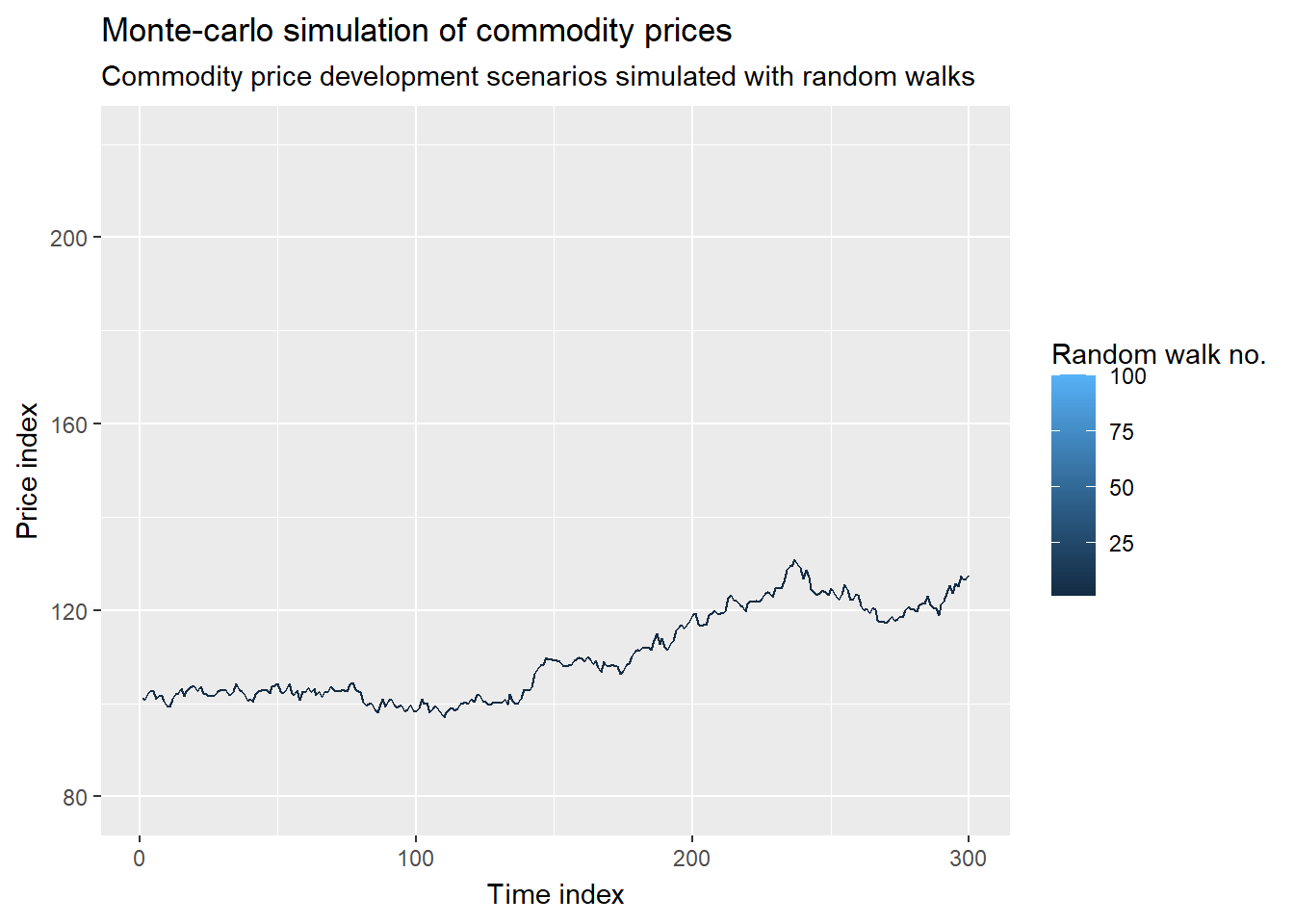# Monte-carlo animation with gganimateIn this article I implement a monte-carlo animation using gganimate in R. In one of my previous posts I introduced monte-carlo simulation. Monte-carlo simulation is one of the widely applied simulation techniques in supply chain management and operations management. I explained how one can assess the risk associated with stock price volatility using monte-carlo simulation. I already back then implemented a monte-carlo simulation in R. I furthermore related it to other relevant simulation techniques such as discrete-event simulation, agent-based simulation, systems dynamics, and gaming.

In this article I will show how you can animate a plot-based visualization of an underlying monte-carlo simulation. I will do so using R and the R-package gganimate. As also described in one of my other posts gganimate is compatible with ggplot2. And, I have also used gganimate to animate ggmap-visualizations in R. ggmap is nothing but an extension of ggplot2, with the core functionality and grammtics being original ggplot2 plots. For this reason both ggplot2 and ggmap are compatible with gganimate.

## Motivation for monte-carlo animations

Monte-carlo simulation is a process based on random distributions and iterative realization of probabilities. I apply monte-carlo simulations whenever an analytical solution to a problem is not that obvious or maybe not even available. This also means that monte-carlo simulation is frequently used to solve complex problems.

A monte-carlo animation can make the simulation easier to understand, as is generally the case and purpose of visualizations. It is furthermore well known from research that visualizations and animations result in more productive meetings, discussions and better ideas. This especially true throughout the exploratory stages of a supply chain analytics project.

## Monte-carlo animation data structure in R

Before any animation can be produced I have to implement the monte-carlo simulation and store its values in a dataframe. I will implement a monte-carlo simulation similar to my example of simulating stock price development using random walks.

I implement 100 simulation runs, all starting from the same starting point. The starting point could be current price of a stock or a commodity (futures or similar)

I store all simulation values, i.e. all values of each simulation run in one and the same dataframe. This dataframe contains the following variables (columns):

• simulation run (run no. 1 to 100)
• time index value (could be days, weeks etc.)
• price index value (could be commodity price in e.g. USD)

The random walk will go on for 300 days, i.e. 300 time indices.

I implement all of this in the coding segment displayed below:

``````# create empty data frame to be populated
data = as.data.frame(matrix(nrow=100*300,ncol=3))
# assign column names to dataframe
colnames(data) = c("run","time","price")
# execute 300-day long random walk, with 100 random walks in total
for(i in 1:100){
price = 100
for(j in 1:300){
run = i
return = rnorm(n=1,mean=0.001,sd=0.01)
time = j
data\$run[(i-1)*300+j] = i
data\$time[(i-1)*300+j] = j
price = price*(1+return)
data\$price[(i-1)*300+j] = price
}
}
# display head of generated simulation dataframe

## Creating ggplot2 visualizations for monte-carlo animation

By now we have generated all data required for plotting the simulation runs in a ggplot2. I will create line plots in different colors.

`````` import relevant libraries
library(ggplot2)
# create a line plot, with one line per random walk ("run")
ggplot(data) +
geom_line(mapping=aes(x=time,y=price,color=run,group=run)) +
labs(title = "Monte-carlo simulation of commodity prices",
subtitle = "Commodity price development scenarios simulated with random walks",
color = "random walk no.") +
xlab("Time index") +
ylab("Price index")``````

## Rendering monte-carlo animation with gganimate

Finally, I can end of this post by generating a ggplot2-based animation, using the R-package gganimate. I save the animation as a .gif-file.

``````# import relevant libraries
library(gganimate)
# create animation
ggplot(data) +
geom_line(mapping=aes(x=time,y=price,color=run,group=run)) +
labs(title = "Monte-carlo simulation of commodity prices",
subtitle = "Commodity price development scenarios simulated with random walks",
color = "random walk no.") +
xlab("Time index") +
ylab("Price index") +
transition_states(run, transition_length = 3, state_length = 1)``````
``````# save animation as gif file
anim_save("montecarloanimation1.gif")``````

I am not satisfied with this animatino yet. I want the animation to add one random walk after the other to the plot, i.e. I want the lines to accumulate. The animation is complete when all random walks have been added. In order for me to achieve this I have to make some minor adjustments to the code. I do so below, resulting in the animation displayed at the bottom.

``````# for every run, add all previous run
data\$cumulrun = data\$run
for(i in 1:100){
if(1 < i){
localdata = data[1:((i-1)*300),]
localdata\$cumulrun = rep(i,times=(i-1)*300)
data = rbind(data,localdata)
}
}
# create animation again
ggplot(data) +
geom_line(mapping=aes(x=time,y=price,color=run,group=run)) +
labs(title = "Monte-carlo simulation of commodity prices",
subtitle = "Commodity price development scenarios simulated with random walks",
color = "random walk no.") +
xlab("Time index") +
ylab("Price index") +
transition_states(cumulrun, transition_length = 3, state_length = 1)``````
``````# save monte carlo animation
anim_save("montecarloanimation2.gif")``````

## Relevant articles related to gganimate, ggplot and ggmap

I list some relevant articles below. They will enable you to apply gganimate to all kinds of 2D animations, not just conventional plots. For example, you can use gganimate to animate map-based visualizations.

## Concluding remarks

In this article I implemented a monte-carlo simulation with gganimate in R. It is compatible with ggplot2 and ggmap. All these three packages in R are free to use and can be configured and adjusted to various use cases.

In other articles, linked to in this post, I e.g. show how to make a map-based animation in R. But you could do any kind of 2D animation with gganimate, ggmap, and ggplot2. For example, you can create custom backgrounds for ggplot2 plots.

Monte-carlo animations are useful because they can convey information, tell a story and make monte-carlo simulations easier to understand. Project that make use of not just analytics but also animations and visualiuation tend to be much more succesful. This is not just a subjective feelling but rather a fact backed by research.